A Source Book in Greek Science

Contents:
Author: Claudius Ptolemy

Trigonometry

# Application of Trigonometry

Ptolemy, Almagest II. 3

Given the length of the longest day, to determine the latitude of a place, and conversely.1

A. Now let it be required, given the length of the longest day, to find the elevation of the pole [i.e., the latitude], that is, arc BZ of the meridian.

On the same figure as before,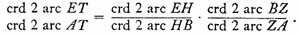But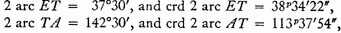Again,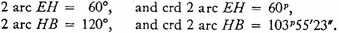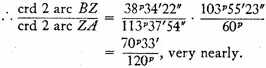very nearly.

Now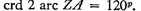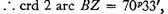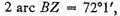and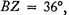very nearly.1

B. Now conversely, let arc BZ, representing, on the same figure, the elevation of the pole, be given. Suppose it is determined by observation to be 36°. Let it be required to find the difference between the shortest or longest day and the day of the equinox, i.e., 2 arc ET.

By the same theorem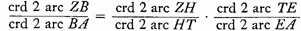But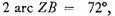and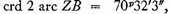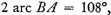2 and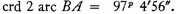Again,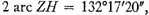3 and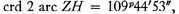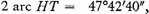4 and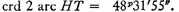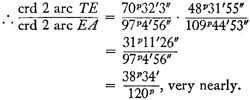But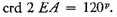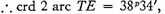and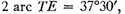very nearly.

This represents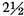equinoctial hours.     Q.E.D.

1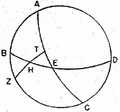The place Ptolemy has in mind is Rhodes, at approximately 36° north latitude, where the length of the longest day is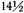hours. In the accompanying figure ABCD is the meridian, BED the horizon, AEC the equator, Z a pole, H the corresponding solstitial point, and T the point where the great circle through Z and H intersects the equator. ET corresponding to half of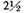hours, measures 18°45′, and AT is its complement; EH has previously been found to be 30°. The equation in both parts is based on Menelaus’s Theorein discussed above, and the computation is performed with the help of the Table of Chords.

1 I.e., a place where the length of the longest day is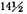hours is at latitude 36°. The equation, in modern terms, is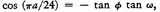where ø, is the latitude of the place, ω the obliquity of the ecliptic, and a the length of the longest day in hours. The maximum value of a in this formula is 24, the length of the longest day at the arctic and antarctic circles. A more complicated formula is necessary for the higher latitudes. The discussion does not take account of (1) the fact that the sun is not a point of light, and (2) the effect of atmospheric refraction in lengthening the time between apparent sunrise and apparent sunset.

2 I.e.,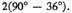3 I.e.,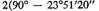[obliquity of the ecliptic]).

4 I.e., double the obliquity of the ecliptic.

Contents:

### Related Resources

Ptolemy

Title: A Source Book in Greek Science

Select an option:

## Email Options

Title: A Source Book in Greek Science

Select an option: